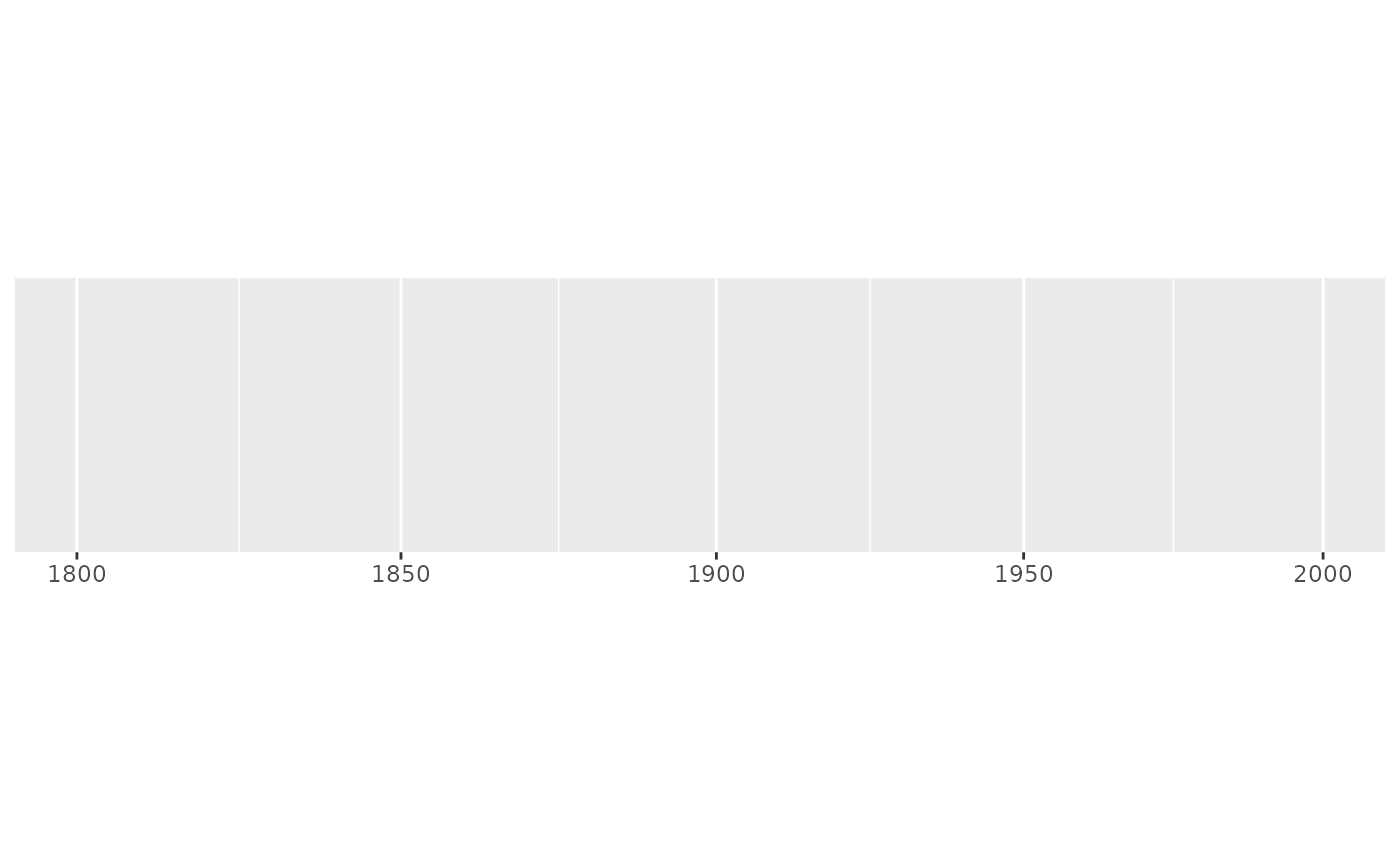This algorithm starts by looking for integer powers of base. If that doesn't provide enough breaks, it then looks for additional intermediate breaks which are integer multiples of integer powers of base. If that fails (which it can for very small ranges), we fall back to extended_breaks()

## Usage

breaks_log(n = 5, base = 10)

## Arguments

n

desired number of breaks

base

base of logarithm to use

## Details

The algorithm starts by looking for a set of integer powers of base that cover the range of the data. If that does not generate at least n - 2 breaks, we look for an integer between 1 and base that splits the interval approximately in half. For example, in the case of base = 10, this integer is 3 because log10(3) = 0.477. This leaves 2 intervals: c(1, 3) and c(3, 10). If we still need more breaks, we look for another integer that splits the largest remaining interval (on the log-scale) approximately in half. For base = 10, this is 5 because log10(5) = 0.699.

The generic algorithm starts with a set of integers steps containing only 1 and a set of candidate integers containing all integers larger than 1 and smaller than base. Then for each remaining candidate integer x, the smallest interval (on the log-scale) in the vector sort(c(x, steps, base)) is calculated. The candidate x which yields the largest minimal interval is added to steps and removed from the candidate set. This is repeated until either a sufficient number of breaks, >= n-2, are returned or all candidates have been used.

## Examples

demo_log10(c(1, 1e5))
#> scale_x_log10()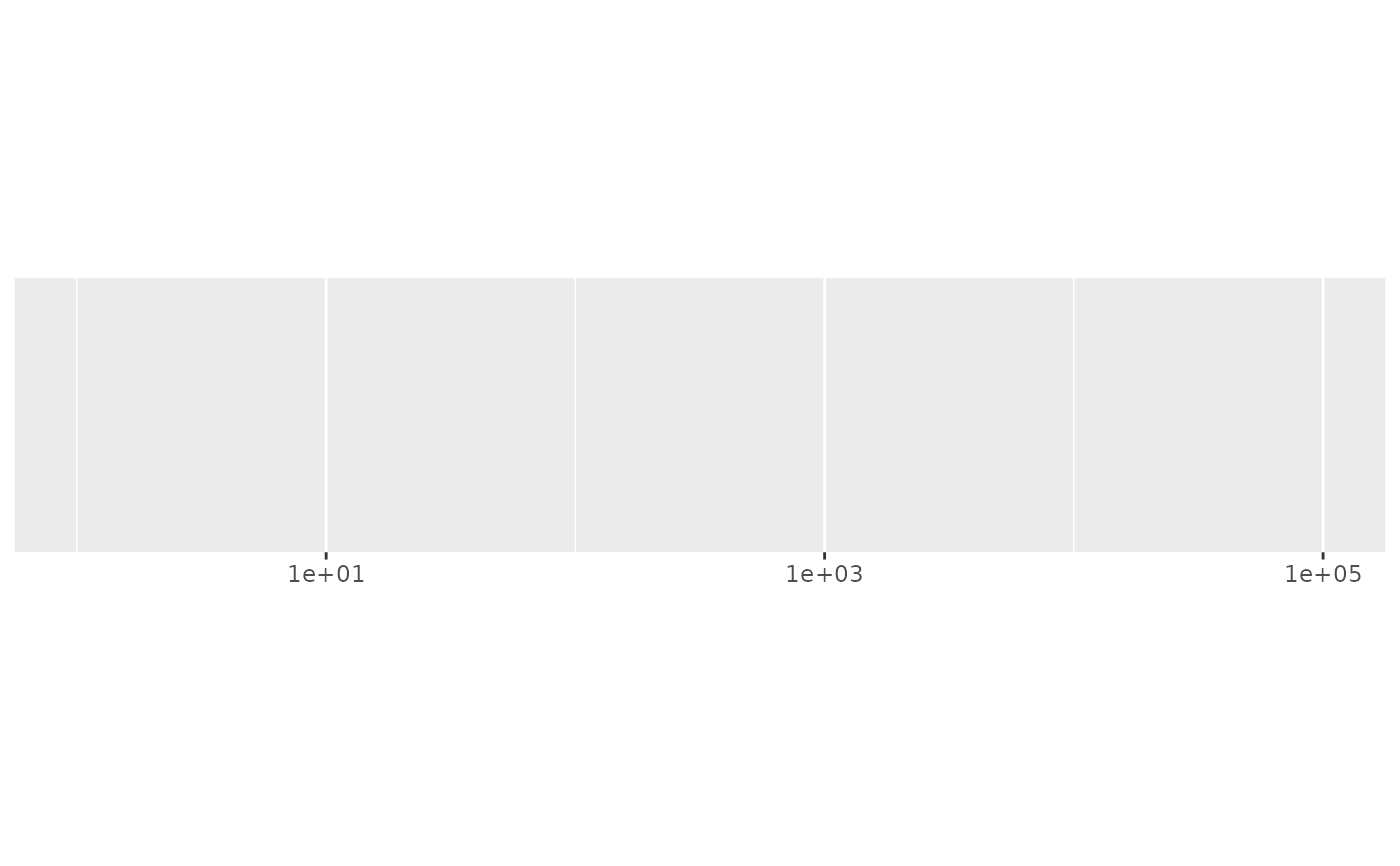demo_log10(c(1, 1e6))
#> scale_x_log10()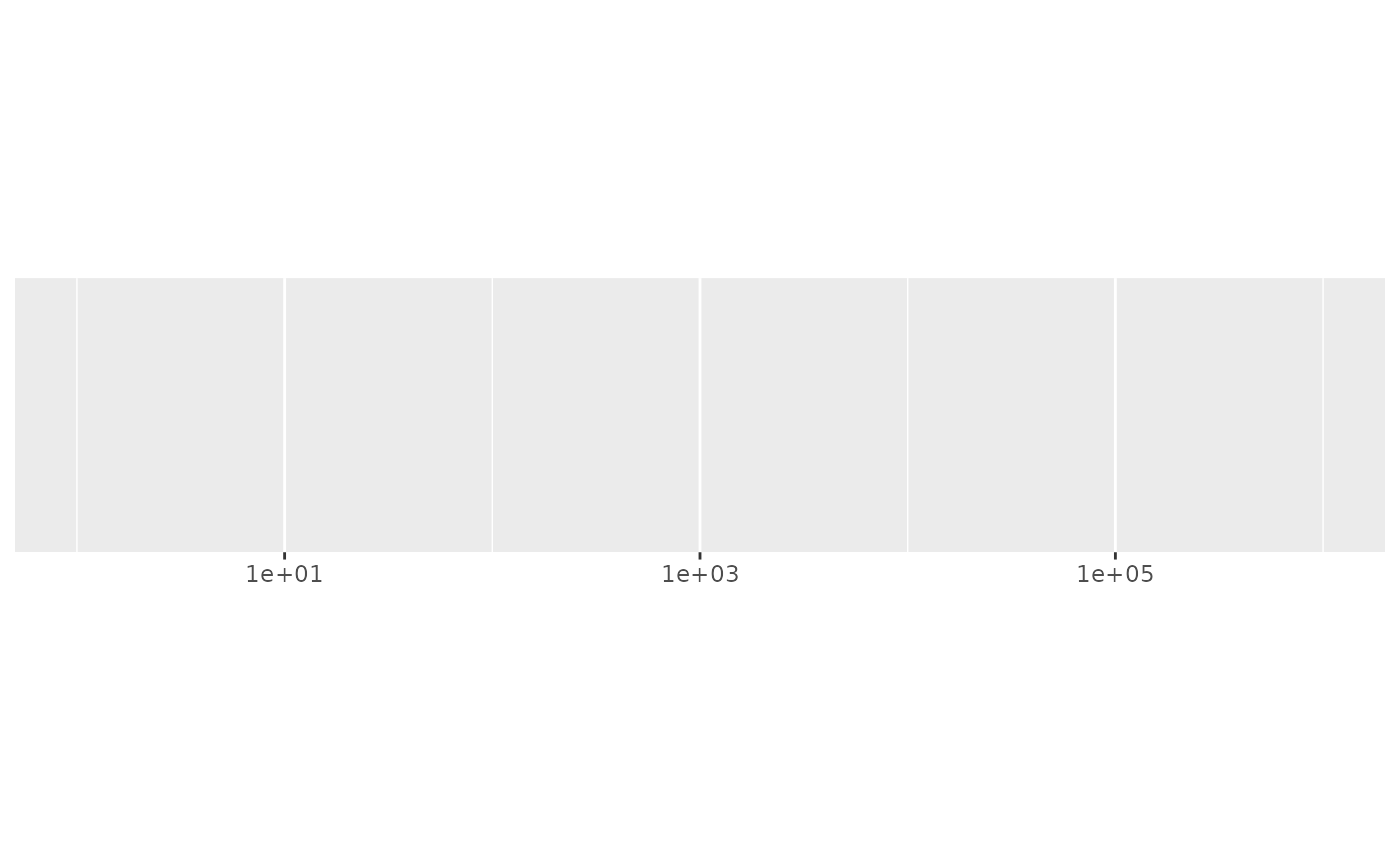# Request more breaks by setting n
demo_log10(c(1, 1e6), breaks = breaks_log(6))
#> scale_x_log10(breaks = breaks_log(6))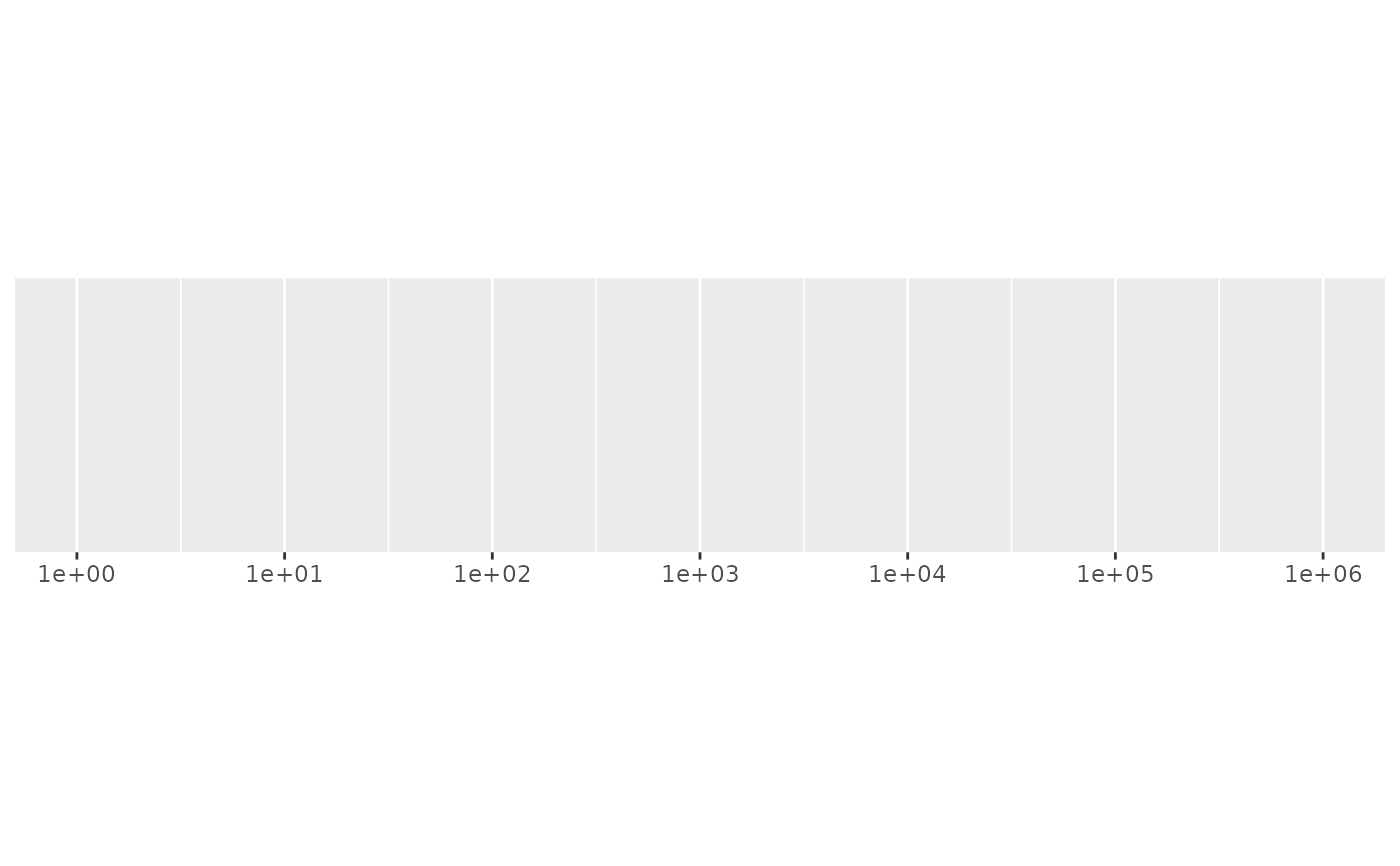# Some tricky ranges
demo_log10(c(2000, 9000))
#> scale_x_log10()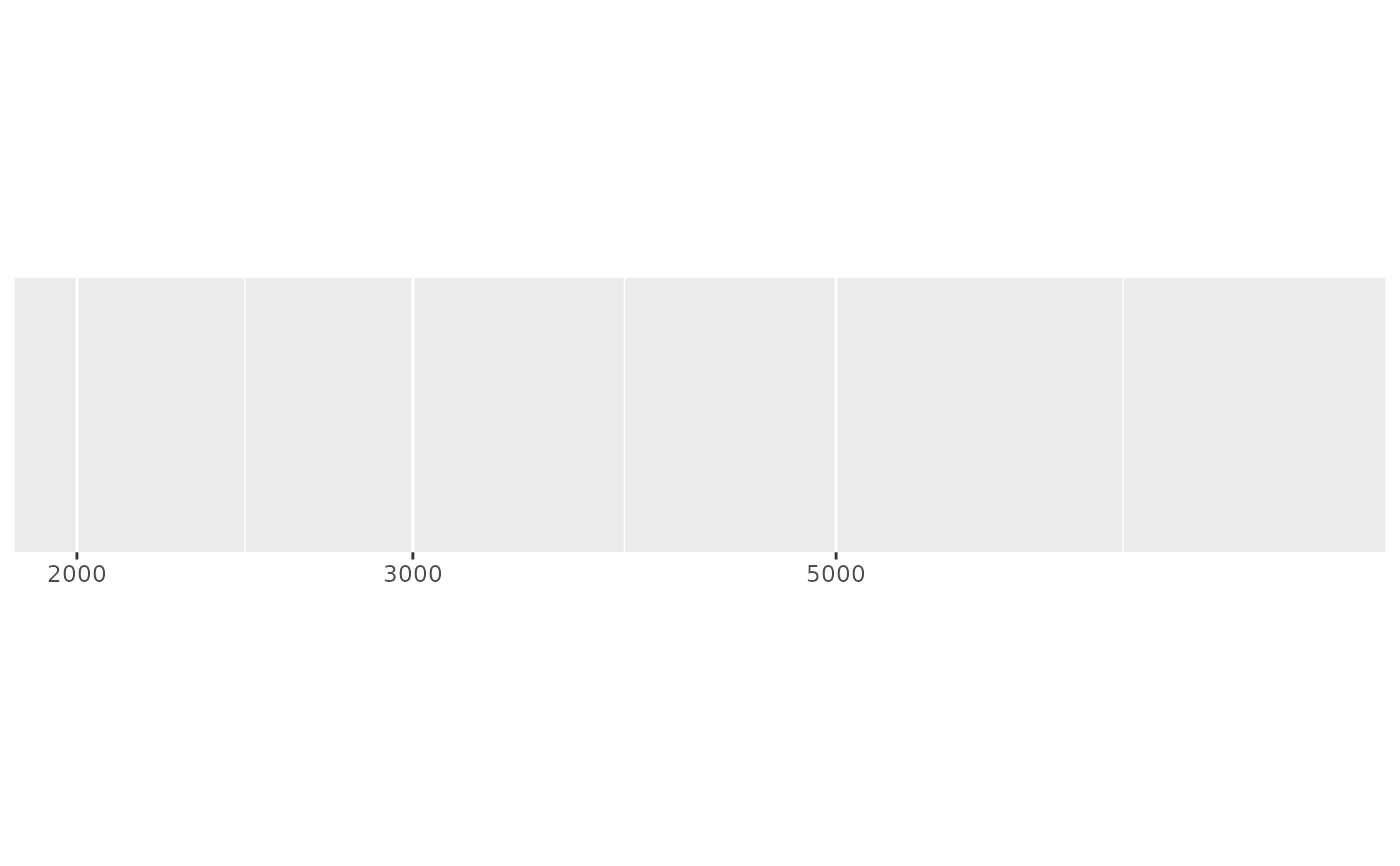demo_log10(c(2000, 14000))
#> scale_x_log10()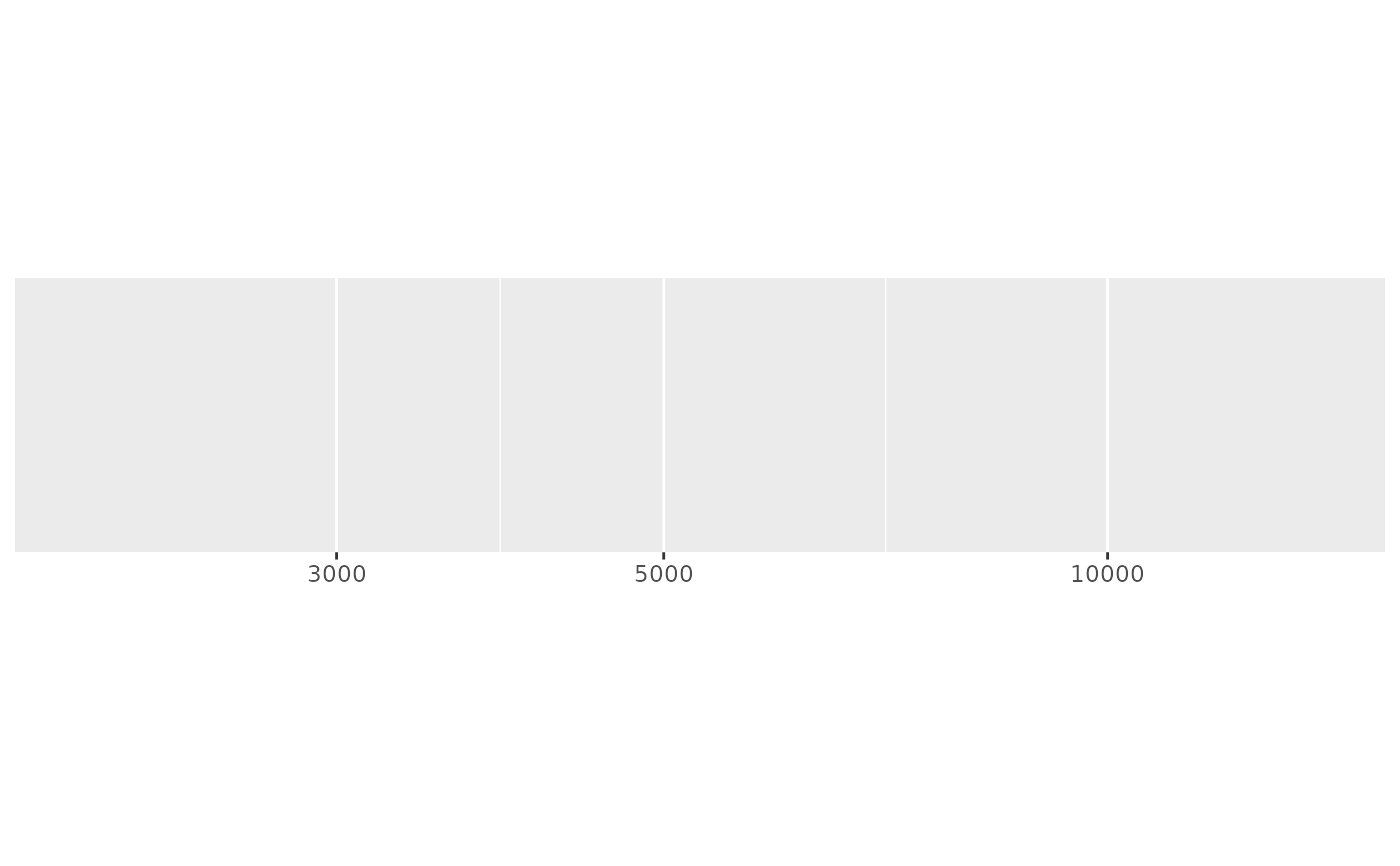demo_log10(c(2000, 85000), expand = c(0, 0))
#> scale_x_log10(expand = c(0, 0))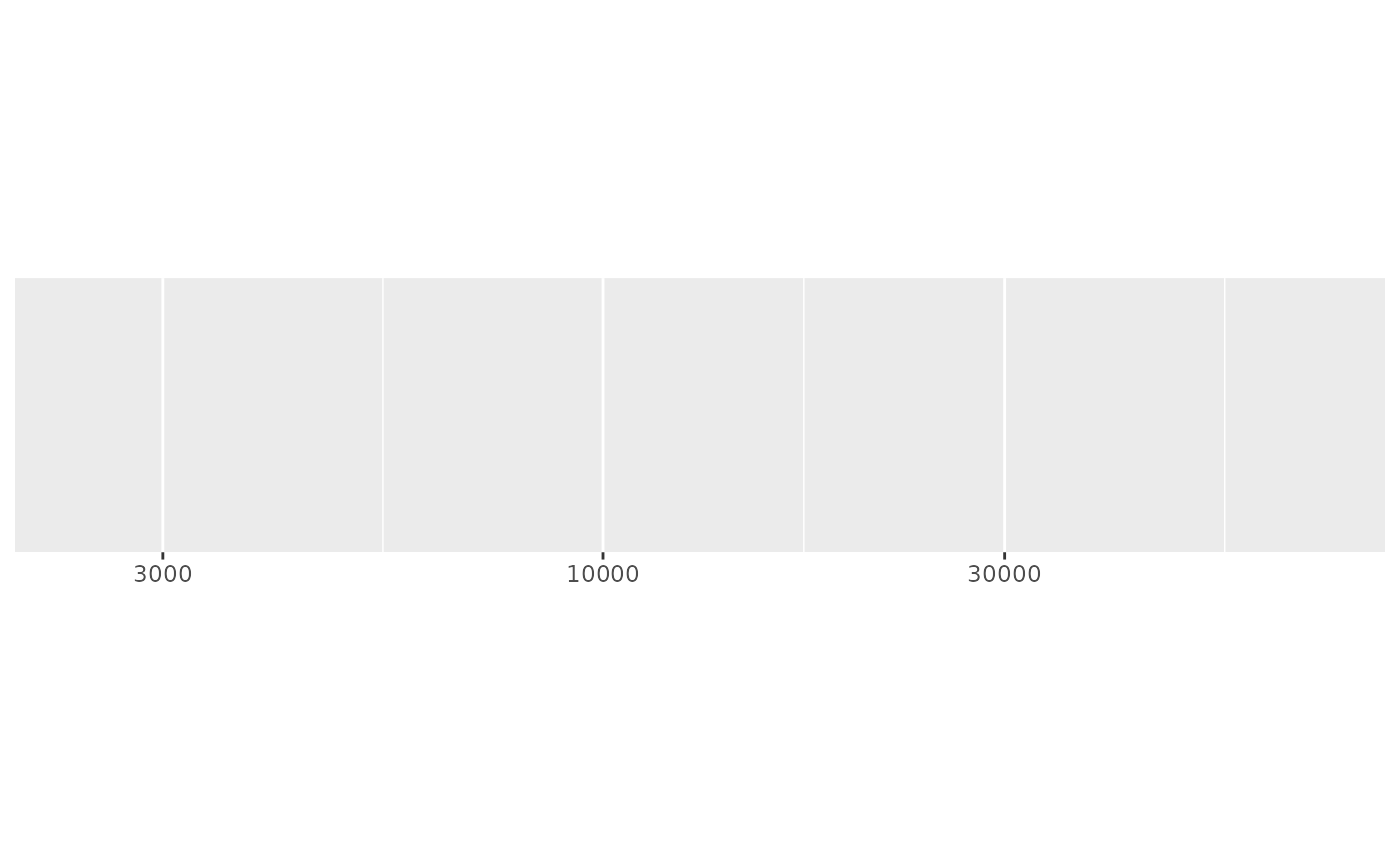# An even smaller range that requires falling back to linear breaks
demo_log10(c(1800, 2000))
#> scale_x_log10()